# 1903021109-方迪琦-Java第三周作业-创建eclipse

 项目 内容 课程班级博客链接 19级信计班（本） 作业要求链接 第三周作业 博客名称 1903021109-方迪琦-java第三周作业-创建eclipse 要求 每到题目要有题目，代码（使用插入代码），截图（只截运行结果）

• 定义三给整型变量a、b、c，题目的初始值分别为4、15、20.对表达式进行运算.
• public class SuanShuDemo{
• public static void mian(String[] args){
• //声明三个整型变量a、b、c
• //分别为a、b、c赋值为4、、15、20
• //计算(a/b)+c的值，并赋值给变量m
• //计算(c%b)*a-c的值，并赋值给变量n
• //输出m和n的值 }}
• 代码
package ff;
public class ff {
public static void main(String[] args) {
// TODO Auto-generated method stub
int a;
int b;
int c;
int m,n;
a=4;
b=15;
c=20;
m=(b/a)+c;
n=(c%b)*a-c;
System.out.println("m="+m);
System.out.println("n="+n);
}

}
• 运行结果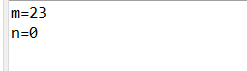• 已知a,b均是整型变量，写出将a,b两个变量中的值互换的程序。（知识点：变量和运算符综合应用）
• 代码
package ff;
public class ff {
public static void main(String[] args) {
// TODO Auto-generated method stub
int a=8 ;
int b=16;
int j=a;
a=b;
b=j;
System.out.println("a = " + a);
System.out.println("b = " + b);
}
}
• 运行结果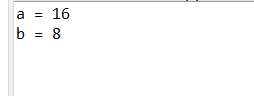• 随意给出一个整数，打印显示他的个位数，十位数，百位数的值。（知识点：取余、除）

输出样例：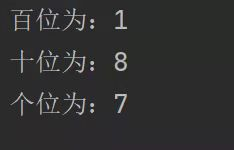• 代码：
package ff;

public class ff {

public static void main(String[] args) {
// TODO Auto-generated method stub
int j = 356;
int 百位数 = j / 100;
int 十位数 = j % 100/10;
int 个位数 = j % 10;
System.out.println("百位输出为：" + 百位数 );
System.out.println("十位输出为：" + 十位数 );
System.out.println("个位输出为：" + 个位数 );
}

}
• 运行结果：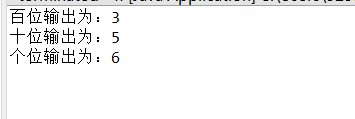• 把摄氏温度37度转换为华氏温度，摄氏温标（C）和华氏温标（F）之间的换算关系为：F=C×1.8+32  C=(F-32)÷1.8
• 代码
package ff;

public class ff {
public static void main(String[] args) {
// TODO Auto-generated method stub
double c;
double f;
c=37;
f=c*1.8+32;
c=(f-32)/1.8;
System.out.println("f=" +f );
}
}
• 运行结果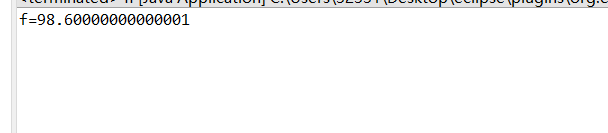1、阅读完《如何创建Java程序》后，原先不明白的步骤已经掌握了；

2、通过Java语言基础上（上），对标识符、关键字需要注意的点得到了巩固；

3、各种数据类型的定义得到了巩固；

4、变量与常量如何定义类型和如何赋值得到了更近一步的认识。

posted @ 2022-03-20 16:08  ~distance~  阅读(29)  评论(0编辑  收藏  举报